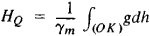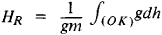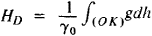Leveling Elevation

The following article is from The Great Soviet Encyclopedia (1979). It might be outdated or ideologically biased.

Leveling Elevation

the elevation of a point on the earth’s surface, determined by methods of leveling, relative to an initial point (point O) selected on the geoid. The elementary difference in elevations dh of two successive points that are relatively close to one another along a leveling line is found by geometric leveling. If the magnitudes of the force of gravity g along the leveling line are known, the leveling elevation of point K relative to point O is determined according to the formulawhere γm is the mean value of the normal force of gravity along segment HQ of the normal toward the surface of the earth ellipsoid, which gives the system of coordinates for studying the figure and gravitational field of the earth. This method of determining the leveling elevation was proposed by M. S. Moloden-skii in 1945. The leveling elevation obtained by this method is called the normal elevation. The normal leveling elevation added to the elevation of the quasigeoid determines the elevation above the earth ellipsoid.

Attempts were formerly made to use direct leveling to derive the orthometric elevation, which is defined according to the formulawhere gm is the average value of the real force of gravity along a plumb line from the surface of the geoid to the earth’s surface at the point K under investigation. This method requires information about the internal structure of the earth and involves hypotheses regarding the density distribution within it.

In some cases leveling elevations are derived in the form of dynamic elevations, determined according to the formulawhere γ0 is an appropriately selected constant value of the force of gravity for the entire given leveling grid.

In processing the results of geometric leveling, sometimes the only magnitude computed is

Δ W = ∫(OK)gth

which is the difference between the potentials of the force of gravity at points K and O and is called the geopotential marker.

REFERENCE

Eremeev, V. F. , and M. I. Iurkina. Teoriia vysot v gravitatsionnom pole Zemli. Moscow, 1972.

M. I. IURKINA